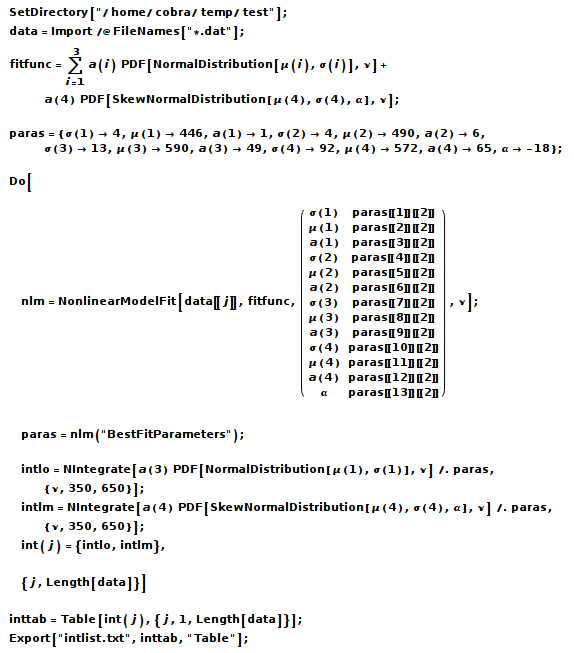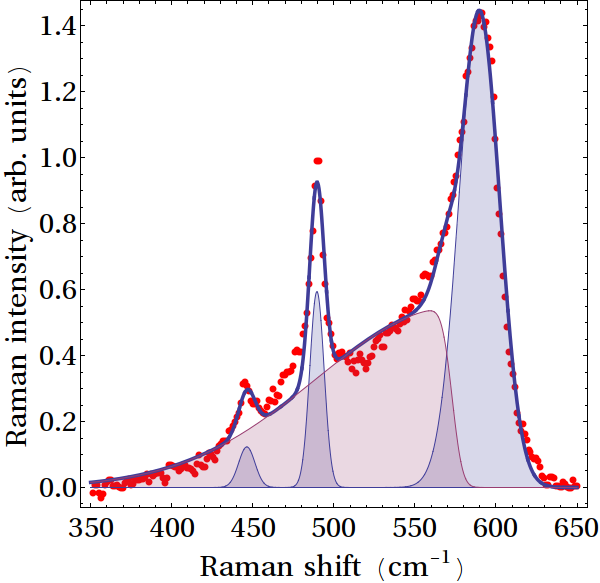# Batch job

What I like about Mathematica is the combination of a stringent and economic code, a decent numerical performance, and the possibility to submit a job remotely via the command line.

Consider the following example:The 2nd line reads in all files having the extension "dat" contained in the directory defined in the 1st line. The array 'data' then contains all data sets as individual elements.

The 3rd line defines a fit function composed of three symmetric and one asymmetric Gaussian conveniently represented by the probability density of appropriate statical distributions. Initial values for all parameters are defined in in the 4th line.

The 5th line starts a loop (concluded in the 11th line) over all data sets. The 6th line defines a least square fit of the data. Note that the initial values are not given as constants, but in form of a declaration that will automatically take into account their change from data set to data set. Hence the 7th line.

In the 8th and 9th line we integrate over individual components of the spectrum. Line 10 defines the result as a list element. The complete list is defined in line 12, and exported in line 13.

My present spectra have 250 points, and the fit and integration for a single spectrum takes about 70 ms. Since I have usually just a few thousand of spectra, the whole business is done in minutes. Plotting the spectra and the fits, by the way, takes significantly longer. The following graph shows an example of such a fit.One can, by the way, generate such graphs even when submitting the calculation from the command line by a "math -noprompt -run "< output &". Just remember to have an Exit[] as the last line in your Mathematica notebook. Then, convert it to InputForm, copy and paste it to you favorite editor, and save it as (for example) batchfit.m.